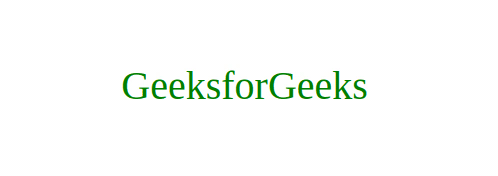# How to spin text on mouse hover using HTML and CSS?The spinning of text on mouse hover is known as the Spin Effect or the Rotation Effect. In this effect, each alphabet of the word is rotated along with any one of the axes (preferably Y-axis). Each word is wrapped inside in <li> tag and then using CSS:hover Selector selector we will rotate each alphabet on Y-axis. We will divide this article into two sections, in the first section we will create the basic structure of the text that will spin. IN the second section we will make that text structure spinnable when the user the hover on that.

Creating Structure: In this section we will create the structure by using HTML.

• HTML Code: In this we have created an unordered-list and wrap each alphabet inside an list-item(li).

 ` ` `<``html` `lang``=``"en"``> ` `    ``<``head``> ` `        ``<``meta` `charset``=``"UTF-8"` `/> ` `        ``<``meta` `name``=``"viewport"`  `              ``content``=``"width=device-width, initial-scale=1.0"` `/> ` `        ``<``title``>Spin Text ` `    `` ` `    ``<``body``> ` `        ``<``ul``> ` `            ``<``li``>G ` `            ``<``li``>e ` `            ``<``li``>e ` `            ``<``li``>k ` `            ``<``li``>s ` `            ``<``li``>f ` `            ``<``li``>o ` `            ``<``li``>r ` `            ``<``li``>G ` `            ``<``li``>e ` `            ``<``li``>e ` `            ``<``li``>k ` `            ``<``li``>s ` `        `` ` `    `` ` ` `

Desingning Structure: In this section we will make that structure spinnable and add the little bit of decoration.

• CSS Code: First we have provide some basic styling like margin, padding and background. Then we have aligned our list-items horizontally using the float property. Finally, use the hover selector to rotate each alphabet along Y-axis on a particular degree. If you want you can use n-th child property to apply some delay to rotation of each alphabet.The use of n-th child comes to personal preferences and needs so if you feel like using it you can surely go for it.

 ` `

Final Solution: It is the combination of the above two codes.

 ` ` `<``html` `lang``=``"en"``> ` `    ``<``head``> ` `        ``<``meta` `charset``=``"UTF-8"` `/> ` `        ``<``meta` `name``=``"viewport"`  `              ``content``=``"width=device-width, initial-scale=1.0"` `/> ` `        ``<``title``>Spin Text ` `        ``<``style``> ` `            ``body { ` `                ``margin: 0; ` `                ``padding: 0; ` `            ``} ` ` `  `            ``ul { ` `                ``padding: 50px; ` `                ``margin: 0; ` `                ``position: absolute; ` `                ``top: 20%; ` `                ``left: 25%; ` `            ``} ` `            ``ul li { ` `                ``list-style: none; ` `                ``color: green; ` `                ``float: left; ` `                ``font-size: 40px; ` `                ``transition: 0.8s; ` `            ``} ` ` `  `            ``ul:hover li { ` `                ``transform: rotateY(360deg); ` `            ``} ` `        `` ` `    `` ` `    ``<``body``> ` `        ``<``ul``> ` `            ``<``li``>G ` `            ``<``li``>e ` `            ``<``li``>e ` `            ``<``li``>k ` `            ``<``li``>s ` `            ``<``li``>f ` `            ``<``li``>o ` `            ``<``li``>r ` `            ``<``li``>G ` `            ``<``li``>e ` `            ``<``li``>e ` `            ``<``li``>k ` `            ``<``li``>s ` `        `` ` `    `` ` ` `

Output:My Personal Notes arrow_drop_upCheck out this Author's contributed articles.

If you like GeeksforGeeks and would like to contribute, you can also write an article using contribute.geeksforgeeks.org or mail your article to contribute@geeksforgeeks.org. See your article appearing on the GeeksforGeeks main page and help other Geeks.

Please Improve this article if you find anything incorrect by clicking on the "Improve Article" button below.